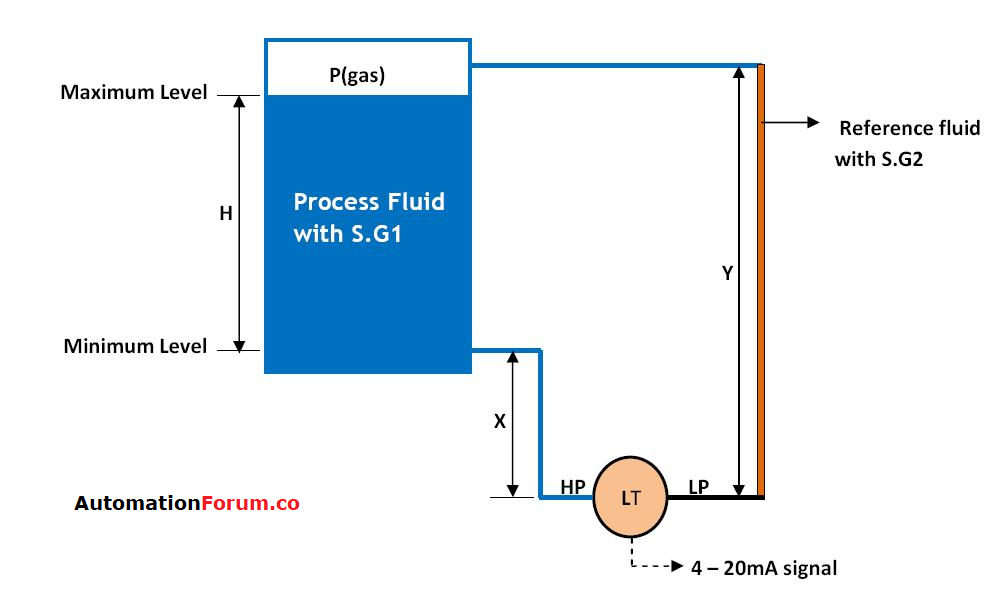Analytical Instrumentation

# What is Zero suppression & Zero Elevation?Zero suppression & Zero elevation are values that correspond to the 4-20mA transmitter range for level measurement using Differential pressure transmitter (DPT).

In signal conditioners, in particular, zero suppression and zero altitudes relate to a reduction (deletion) or angle (altitude) of the nominal “zero” yield so that it is not at zero volts (or milliamps).

### Where Zero elevation & Zero suppression?

The aim of calibration elevation & suppression is to add differential pressure to circumstances where a 4 mA output is required at a point other than 0 differential pressure.

A typical implementation for this is where a device producing a +/- 10-volt signal is to be used and this signal is to be taken into an input of 0-10 volts. You would need to “elevate” the zero point to + 5 volts and rescale the variety to fit within 0 to 10 in order to use the complete assessment variety.

### Zero suppression:

Consider a closed tank, level of liquid in it will be measured using Differential pressure transmitter.

If you mount the transmitter below the tank’s datum line, when the High-end impulse line fills up, the transmitter signal will exceed 4 mA and this will result in a zero error relative to the real zero level of the tank. So that head pressure must be suppressed. By changing the signal to 4 ma. This is the suppression of zero.

### Zero Elevation:

If the transmitter is mounted above the datum line, the signal must be raised from 4 ma to prevent a mistake when the hi-side impulse range is filled. This is a zero elevation.

if you are using a transmitter with HART protocol you can do this just by changing the cal range using the HART communicator